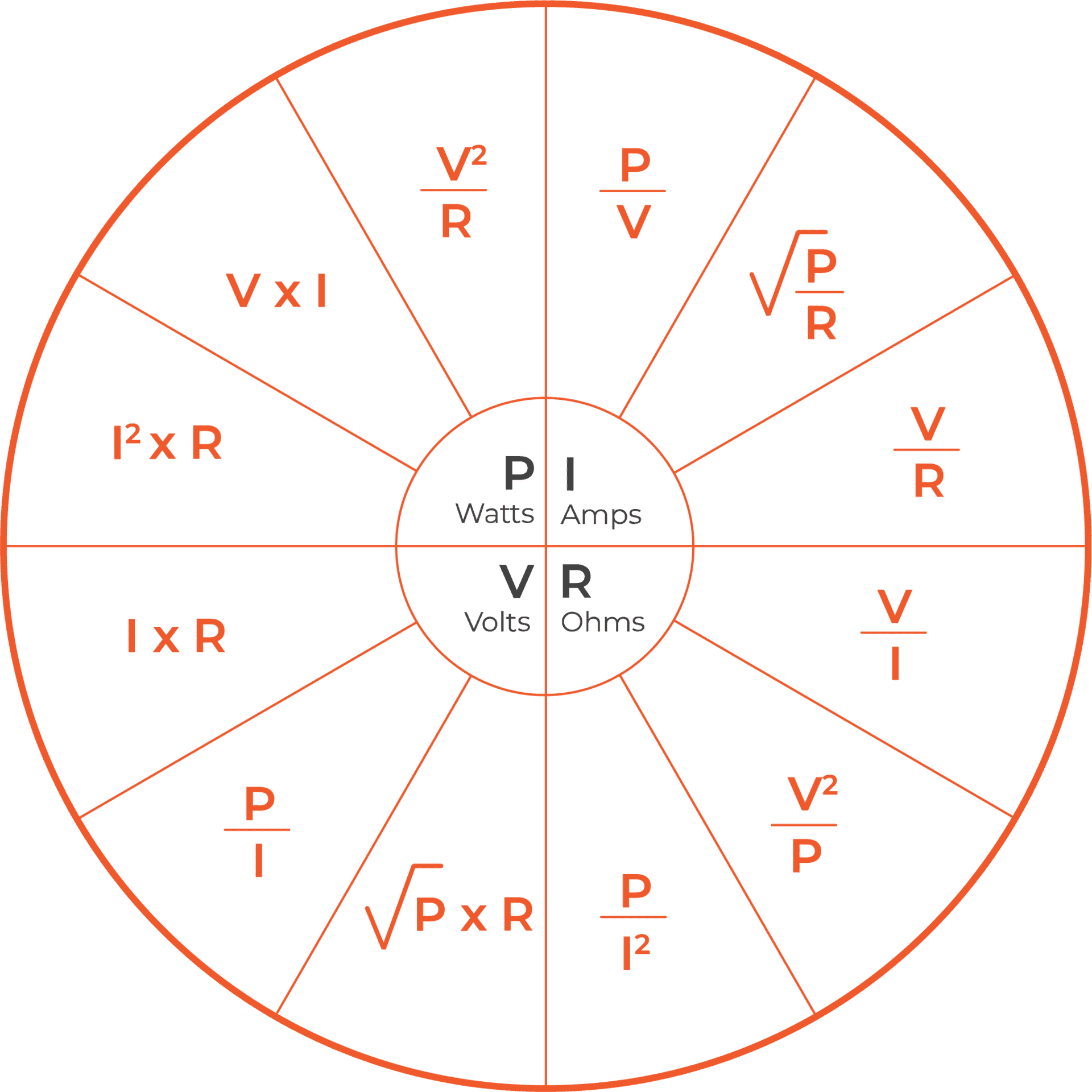# Ohm’s Law Calculator

Voltage:
V
Current:
A
Resistance:
Ω
Power:
WDiscovered by George Simon Ohm, a German physicist in 1827, Ohm’s law explains the relationship between voltage, current, and resistance in an electrical circuit. Now considered one of the basic laws of physics, it states that the current through a conductor between two points is directly proportional to the potential difference across the two points.

Conversions can sometimes be easy if you are talking about the same unit such as micrograms to grams. Other times conversions can be much more difficult like converting between metric and imperial units such as kilograms to ounces. Suntsu has created a weight unit converter to assist you. Our converter can help you convert between the following units:

An electric circuit is a closed circuit that allows an electrical current to flow. The continuous flow of electrical charges (electrons or electron-deficient atoms) through conductors is called a current. The force that influences the charge to flow between two points in an electrical field is known as voltage. Current is met with opposition when it moves through an electric current. That friction is known as resistance. Resistance and voltage are quantities that are always relative between two points. Additionally, the relationship between voltage and current is constant. Mathematically, the law states that V = IR, where: